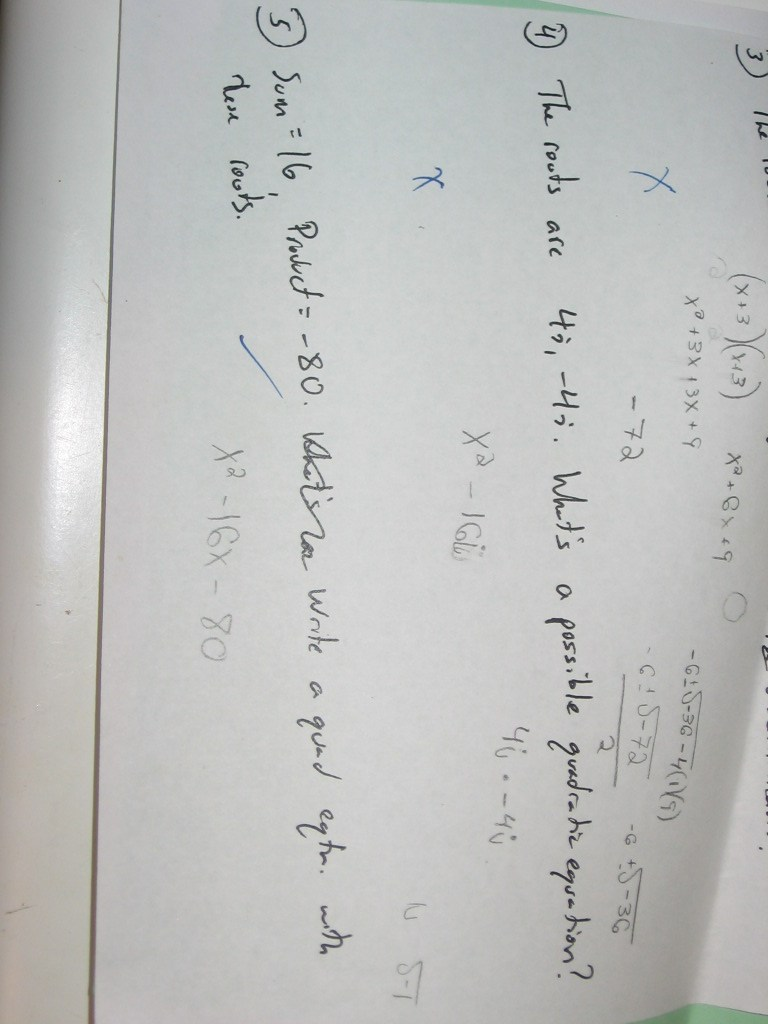Categories

# From complex roots to a quadratic equationHow did this student end up with the answer he did?  What does it seem that he knows about complex numbers?

## 2 replies on “From complex roots to a quadratic equation”

louisesays:

Well, the student does know how to do the difference of two squares. He needs to work on$i^2$ being equal to negative 1 instead of i

khanhdangsays:

I dont think that him not knowing the value of i^2 was the main problem. I actually think that the student had no idea they were suppose to factor this out or else they would have showed some type of factoring method to get where they were. the student should have known that 4i and -4i came from (x+4i)(x-4i) to foil and find the quadratic equation.